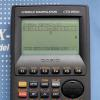# Trigonometric bug in the newest scientific Casio calculator (FX-991EX)

calculator FX-991EX trigonometric newest new trig trigonometry casio bug scientific

3 replies to this topic

### #1jungingj

jungingj

Newbie

•• Members
•• 1 posts

Posted 16 October 2017 - 04:55 PM

I bought a new Casio FX-991EX today and tried to calculate some simple trigonometric functions.

When given:

16*cos(x)^6 - 8*cos(x)^4 - 16*cos(x)^4 + 10*cos(x)^2 - cos(x)^2

the result is:

-1.8*10^(-13)

16*[cos(x)^4]*[cos(x)^2] - 8*cos(x)^4 - 16*cos(x)^4 + 10*cos(x)^2 - cos(x)^2

the result is:

0

16*cos(x)^6 - 8*cos(x)^4 - 16*cos(x)^4 + 9*cos(x)^2

the result is:

0

The true value is 0.
All three expression are equivalent from the algebraic point of view.
I got the same results when I put x=30 (In Deg), so it is not Deg/Rad problem.
I got the same results when I used the FX-82ES.
I do not own anymore scientific calculators so I can not investigate this issue further.

I know that in this forum there are people that are more expert in Casio calculators than I am.
I want to hear your opinions.
thanks.

Edited by jungingj, 16 October 2017 - 05:00 PM.

### #2anon34

anon34

Casio Freak

•• Members
•• 268 posts

Posted 17 October 2017 - 08:04 AM

Those calculator use binary-coded floating point with 15 decimal digits of accuracy, so numerical errors are unavoidable.

Edited by user202729, 17 October 2017 - 08:04 AM.

• jungingj likes this

### #3pan.gejt

pan.gejt

Casio Freak

•• Members
•• 244 posts
• Gender:Male
• Location:CZ

• Calculators:
.

Posted 18 October 2017 - 06:23 AM

I tried the 1st expression

a ) TI-30X Pro ( equivalent of Casio fx-991 derivates) - computing is not possible due to entry-limit (no additional characters can be entered)

b ) HP-35s: 9×10^-12

c ) Sharp EL-W506X: 0

Two graphic calcs

d ) TI-84 Plus CE-T - the result is 0

e ) Casio fx-CG50 - the result is 0

So the conclusion is the result has nothing to do with specific calculator brands, but rather with numeric precision (accuracy) on cheaper calcs. It seems it's HW related together with implemented algorithms.

Edited by pan.gejt, 18 October 2017 - 06:34 PM.

• jungingj likes this

### #4frankmar98

frankmar98

Casio Freak

•• Moderator
•• 127 posts
• Gender:Male
• Location:Spain
• Interests:Science, programming

• Calculators:
CFX-9970G
Graph 90+E (fx-GC50)
fx-9860G SD
HP Prime
TI-84+ CE-T
x2 TI-83+
TI-81
fx-4800p, fx-3650PII
fx-991SPX, fx-991ES PLUS, fx-100W

Posted 10 May 2018 - 11:52 AM

Tested on my CFX-9970G (top of the range Casio calculator in 1999):

-1.7E+1

If I put the parenthesis like this (cos 30)^4 instead of cos(30)^4, the result is:

0.0000 (Sci4 mode)

The old linear input of the CFX and AFX series is different than the new calcs inputs. If you add a function, the calculator won't add parenthesis, a function without parenthesis has more priority than exponents, but less priority than parenthesis.

### Also tagged with one or more of these keywords: calculator, FX-991EX, trigonometric, newest, new, trig, trigonometry, casio, bug, scientific

#### 0 user(s) are reading this topic

0 members, 0 guests, 0 anonymous users